# ML Aggarwal Solutions Class 10 Maths Chapter 4 Linear Inequations

1. Solve the inequation, 3x – 11 < 3 where x ∈ {1,2,3,……,10}. Also, represent its solution on a number line.
Solution:
We have given that
3x – 11 <3
3x < 3 + 11
3x <14
∴x <14/3
But, x ∈ {1,2,3,……,10}
Hence, the solution set is {1,2,3,4}.
The solution is representing on number line: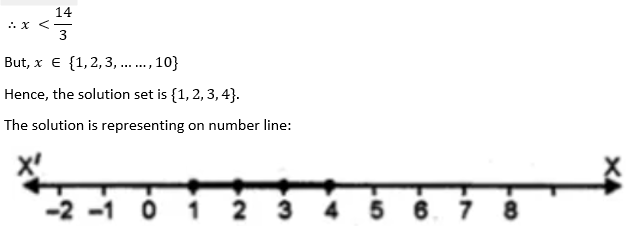2. Solve 2(x – 3) < 1,x ∈ {1,2,3,….10}
Solution:
We have given that,
2(x – 3) < 1
2x – 6 < 1
2x < 7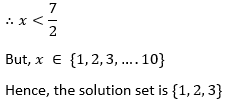3. Solve 5 – 4x > 2 – 3x,x ∈ W. Also represent its solution on the number line.
Solution:
We have given that,
5 – 4x > 2 – 3x
– 4x + 3x > 2 – 5
-x > -3
On multiplying both sides by -1, the inequality reverses
∴x < 3
But, x ∈ W
The solution set is {0,1,2}

The solution is representing on number line:4. List the solution set of 30 – 4 (2x – 1) < 30, given that x is a positive integer.
Solution:
We have given that,
30 – 4 (2x – 1) < 30
30 – 8x + 4 < 30
34 – 8x < 30
-8x < 30 – 34
-8x < -4
On multiplying both sides by -1, the inequality reverses
8x > 4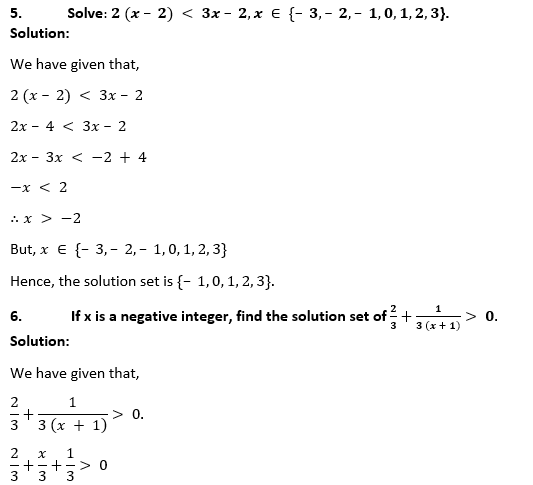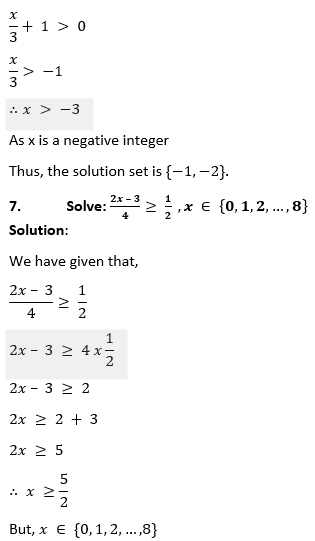Hence, the solution set is {3,4,5,6,7,8}.

8. Solve x – 3 (2 + x) > 2 (3x – 1),x ∈ { – 3,– 2,– 1,0,1,2,3}. Also represent its solution on the number line.
Solution: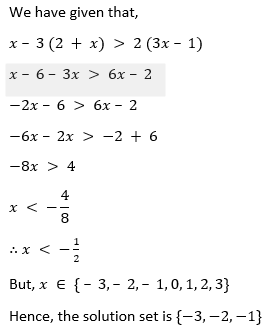9. Given x ∈ {1,2,3,4,5,6,7,9} solve x – 3 < 2x – 1.
Solution:
We have given that,
x – 3 < 2x – 1
x – 2x < – 1 + 3
-x < 2
∴ x > -2
But, x ∈ {1,2,3,4,5,6,7,9}
Hence, the solution set is {1,2,3,4,5,6,7,9}.

10. Given A = {x: x ∈ I,– 4 ≤ x ≤ 4}, solve 2x – 3 < 3 where x has the domain A. Graph the solution set on the number line.
Solution:
We have given that,
2x – 3 < 3
2x< 3+3
2x < 6
∴ x < 3
But x has the domain A = {x: x ∈ I,– 4 ≤ x ≤ 4}
A = {-4,-3,-2,-1,0,1,2,3,4}
Hence, the solution set is {-4,-3,-2,-1,0,1,2}.
The solution is representing on number line: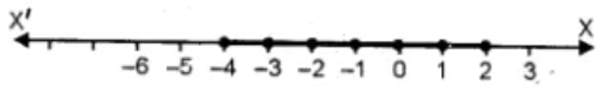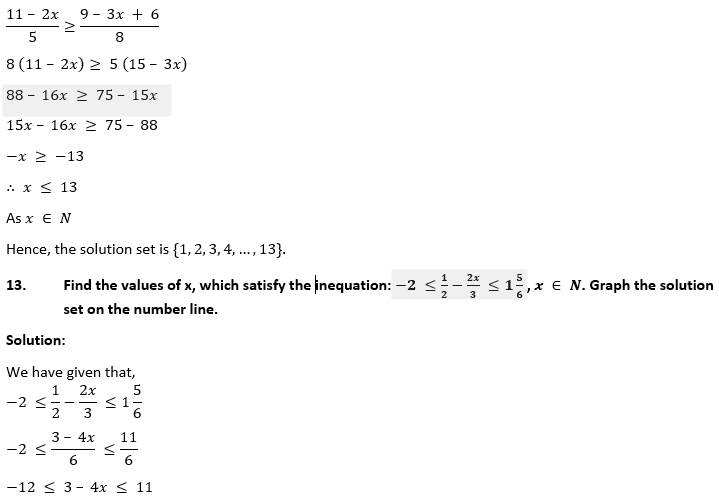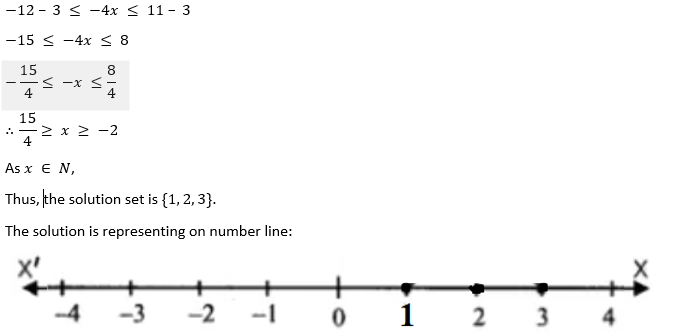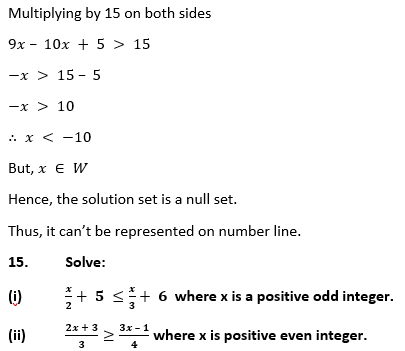Solution:
(i) x is a positive odd integer
We have given that,8x + 12 ≥ 9x – 3
-9x + 8x ≥ -12 – 3
-x ≥ -15
∴ x ≤ 15
As x is positive even integer.
Hence, the solution set is {2,4,6,8,10,12,14}.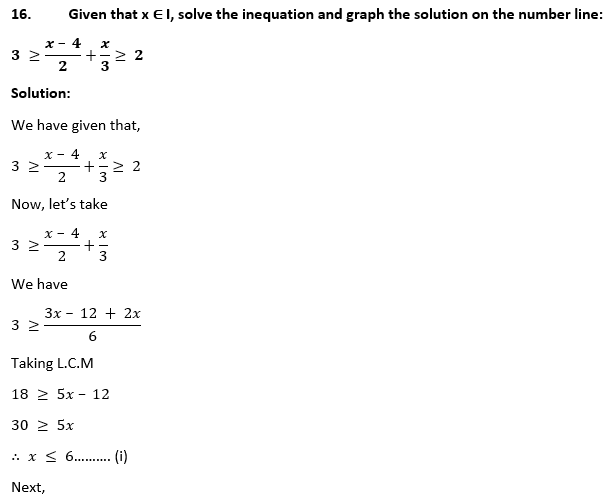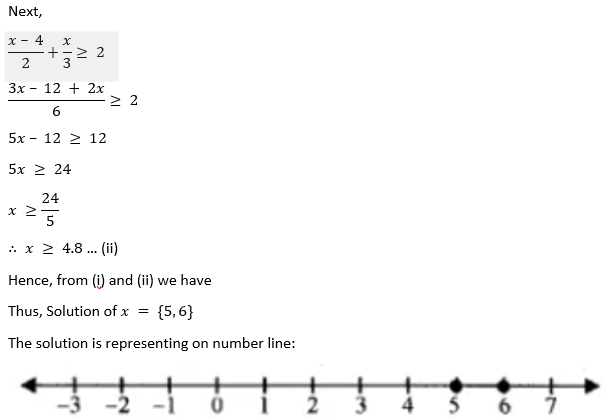17. Given x ∈ {1,2,3,4,5,6,7,9}, find the values of x for which -3 < 2x – 1 < x + 4.
Solution:
We have given that,
-3 < 2x – 1 < x + 4
Now, we have
-3 < 2x – 1 and 2x – 1 < x + 4
-2x < 3 – 1 and 2x – x < 4 + 1
-2x < 2 and x < 5
∴x > -1 and x < 5
As x ∈ {1,2,3,4,5,6,7,9}
Thus, The solution set is {1,2,3,4}.

18. Solve: 1 ≥ 15 – 7x > 2x – 27,x ∈ N
Solution:
We have given that,
1 ≥ 15 – 7x > 2x – 27,
Now, we have
1 ≥ 15 – 7x and 15 – 7x > 2x – 27
7x ≥ 15 – 1 and -2x – 7x > -27 – 15
7x ≥ 14 and -9x > -42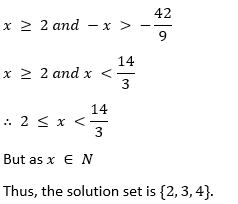19. If x ∈ Z,solve 2 + 4x < 2x – 5 ≤ 3x. Also represent its solution on the number line.
Solution:
We have given that,
2 + 4x < 2x – 5 ≤ 3x
Now, we have
2 + 4x < 2x – 5 and 2x – 5 ≤ 3x
4x – 2x < -5 – 2 and 2x – 3x ≤ 5
2x < -7 and -x ≤ 5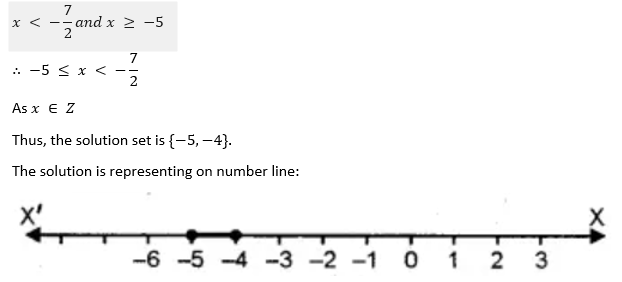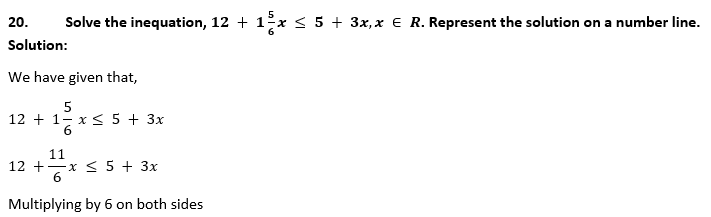72 + 11x ≤ 30 + 18x
11x – 18x ≤ 30 – 72
-7x ≤ -42
-x ≤ -6
∴x ≥ 6
As x ∈ R
Thus, The solution set is {x∶ x ∈ R,x ≥ 6}
The solution is representing on number line: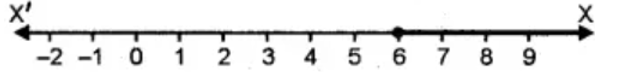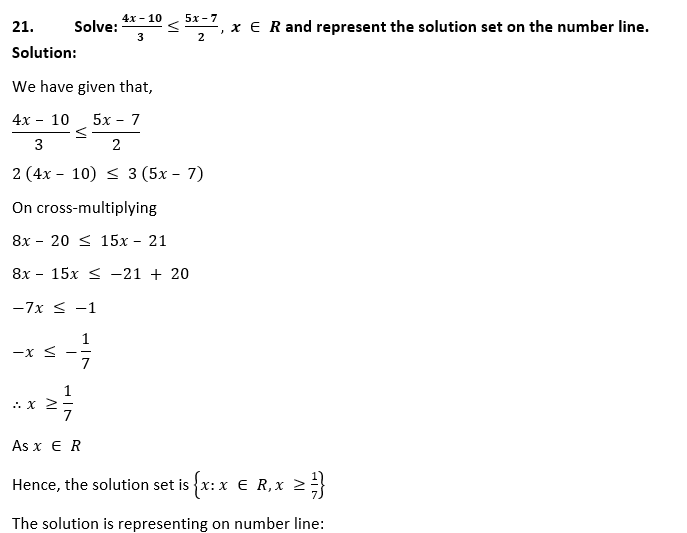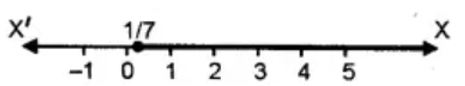23. Solve the inequation – 3 ≤ 3 – 2x < 9,x ∈ R. Represent your solution on a number line.
Solution:
We have given that,
– 3 ≤ 3 – 2x < 9
– 3 – 3 ≤ – 2x < 9 – 3
-6 ≤ -2x < 6
-3 ≤ -x < 3
∴-3 < x ≤ 3
As x ∈ R
The solution set is {x: x ∈ R,-3 < x ≤ 3}
The solution is representing on number line:

24. Solve 2 ≤ 2x – 3 ≤ 5,x ∈ R and mark it on a number line.
Solution:
We have given that,
2 ≤ 2x – 3 ≤ 5
2 + 3 ≤ 2x ≤ 5 + 3
5 ≤ 2x ≤ 8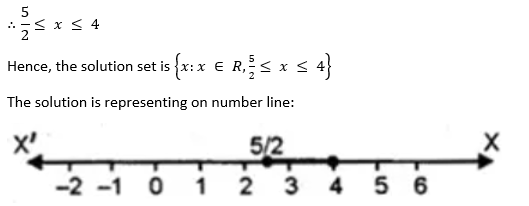25. Given that x ∈ R, solve the following inequation and graph the solution on the number line: -1 ≤ 3 + 4x < 23.
Solution:
We have given that,
-1 ≤ 3 + 4x < 23
-1 – 3 ≤ 4x < 23 – 3
-4 ≤ 4x < 20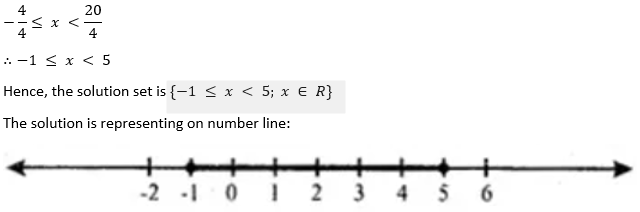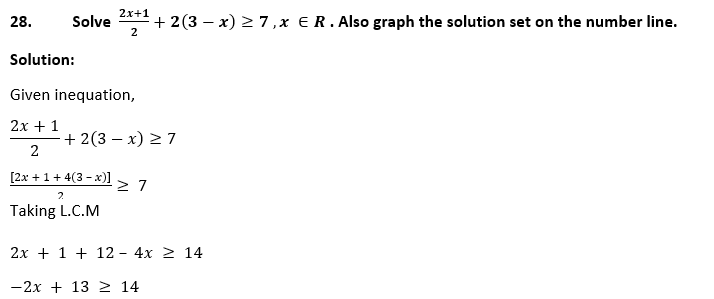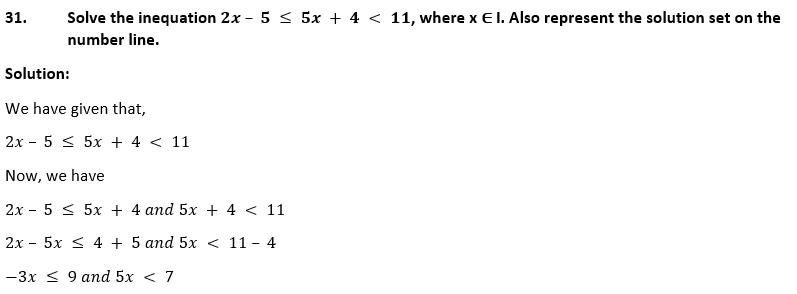32. If x ∈ I,A is the solution set of 2 (x – 1) < 3x – 1 and B is the solution set of 4x – 3 ≤ 8 + x, find A ∩ B.
Solution:
We have given that,
2 (x – 1)< 3x – 1 and 4x – 3 ≤ 8 + x for x ∈ I
Solving for both, we have
2x – 3x < 2 – 1 and 4x – x ≤ 8 + 3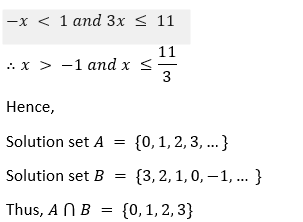33. If P is the solution set of -3x + 4 < 2x – 3,x ∈ N and Q is the solution set of 4x – 5 < 12,x ∈ W, find
(i) P ∩ Q
(ii) Q – P.
(ii) Q – P.
Solution:
We have given that,
-3x + 4 < 2x – 3 where x ∈ N and 4x – 5 < 12 where x ∈ W
So, solving
-3x + 4 < 2x – 3 where x ∈ N
-3x – 2x < -3 – 4
-5x < -7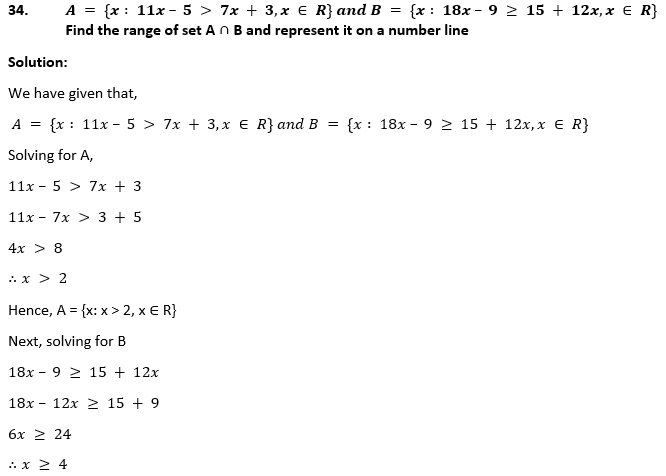Hence, B = {x : x ≥ 4, x ∈ R}
Thus, A ∩ B = x ≥ 4
The solution is representing on number line: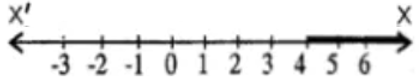35. Given: P {x∶ 5 < 2x – 1 ≤ 11,x ∈ R}
Q {x∶ – 1 ≤ 3 + 4x < 23,x ∈ I} where
R = (real numbers), I = (integers)
Represent P and Q on number line. Write down the elements of P ∩ Q.
Solution:
Given, P {x∶ 5 < 2x – 1 ≤ 11,x ∈ R} and Q {x∶ – 1 ≤ 3 + 4x < 23,x ∈ I}
Solving for P,
5 < 2x – 1 ≤ 11
5 + 1 < 2x ≤ 11 + 1
6 < 2x ≤ 12
∴3 < x ≤ 6
Hence, P = P {x∶ 3 < x ≤ 6,x ∈ R}
The solution is representing on number line: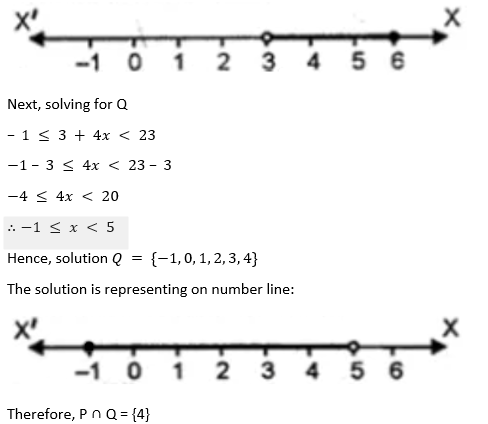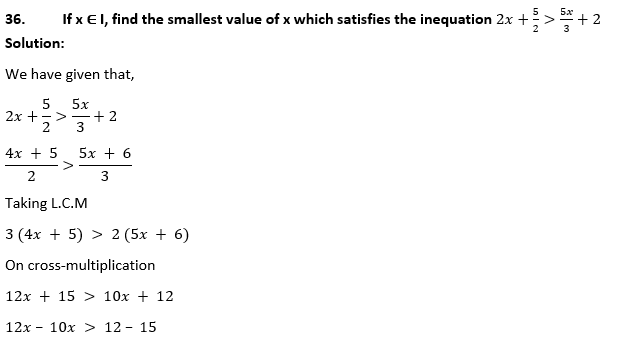2x > -3
∴x > -3/2
Hence, for x ∈ I the smallest value of x is -1.

37. Given 20 – 5 x < 5 (x + 8), find the smallest value of x, when
(i) x ∈ I
(ii) x ∈ W
(iii) x ∈ N.
Solution:
We have given that,
20 – 5 x < 5 (x + 8)
20 – 5x < 5x + 40
-5x – 5x < 40 – 20
-10x < 20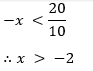Thus,
(i) For x ∈ I, the smallest value = -1

(ii) For x ∈ W, the smallest value = 0

(iii) For x ∈ N, the smallest value = 1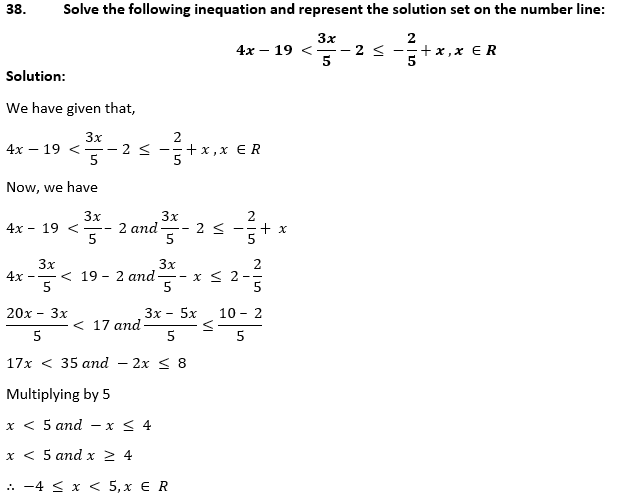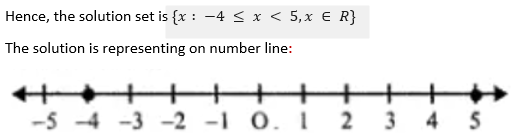39. Solve the given inequation and graph the solution on the number line:
2y – 3 < y + 1 ≤ 4y + 7;y ∈ R.
Solution:
We have given that,
2y – 3 < y + 1 ≤ 4y + 7
Now, we have
2y – 3 < y + 1 and y + 1 ≤ 4y + 7
2y – y < 1 + 3 and y – 4y ≤ 7 – 1
y < 4 and -3y ≤ 6
y < 4 and -y ≤ 2 ⇒ y ≥ -2
∴ -2 ≤ y < 4
The solution set is {y : -2 ≤ y < 4, y ∈ R}
The solution is representing on number line: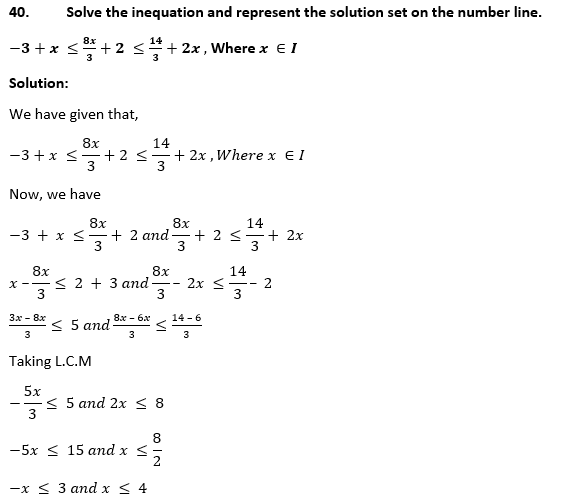x ≥ -3 and x ≤ 4
∴ -3 ≤ x ≤ 4
Thus, the solution set is {-3, -2, -1, 0, 1, 2, 3, 4}
The solution is representing on number line: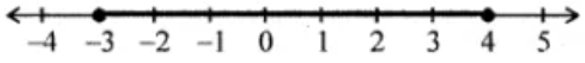41. Find the greatest integer which is such that if 7 is added to its double, the resulting number becomes greater than three times the integer.
Solution:
Let’s consider the greatest integer to be x
Then according to the given condition,
we have
2x + 7 > 3x
2x – 3x > -7
-x > -7
∴x < 7 ,x ∈ R
Hence, the greatest integer value is 6.

42. One-third of a bamboo pole is buried in mud, one-sixth of it is in water and the part above the water is greater than or equal to 3 metres. Find the length of the shortest pole.
Solution:
Let’s assume the length of the shortest pole = x metre
Now,Multiplying by 6
Therefore, the length of the shortest pole is 6 metres.

CHAPTER TEST

1. Solve the inequation: 5x – 2 ≤ 3 (3 – x) where x ∈ {-2, -1, 0, 1, 2, 3, 4}. Also represent its solution on the number line.
Solution:
We have given that,
5x – 2 ≤ 3 (3 – x)
5x – 2 ≤ 9 – 3x
5x + 3x ≤ 9 + 2
8x ≤ 11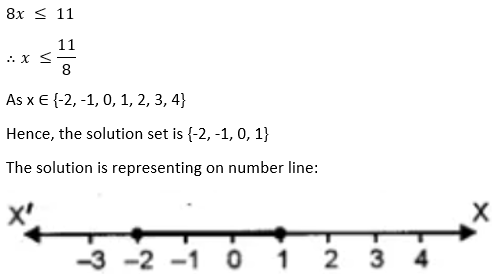2. Solve the inequation: 6x – 5 < 3x + 4,x ∈ I
Solution:
We have given that,
6x – 5 < 3x + 4
6x – 3x < 4 + 5
3x < 9
x <9/3
∴x < 3
As x ∈ I
Hence, the solution set is {2, 1, 0, -1, -2, …}

3. Find the solution set of the inequation x + 5 ≤ 2x + 3; x ∈ R
Graph the solution set on the number line.
Solution:
We have given that,
x + 5 ≤ 2x + 3
x – 2x ≤ 3 – 5
-x ≤ -2
∴x ≥ 2
As x ∈ R
Hence, the solution set is {2, 3, 4, 5, …}
The solution is representing on number line: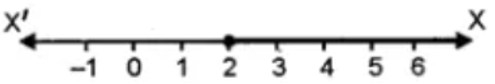4. If x ∈ R (real numbers) and -1 < 3 – 2x ≤ 7, find solution set and present it on a number line.
Solution:
We have given that,
-1 < 3 – 2x ≤ 7
-1 – 3 < -2x ≤ 7 – 3
-4 < -2x ≤ 4